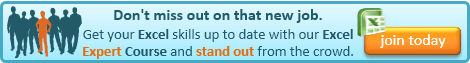From the Not Just Numbers blog:

When working with financial transactions, we often want to analyse them between our financial periods rather than just calendar months.

This is pretty easy if your financial year is January to December and  your periods are the calendar months, but what if your year starts in April or you have four and five week periods – or both?

I’ll explain how to address each of these scenarios in this post.

In each case, let us assume the date we want to identify the period for is held in cell A2.

Calendar Year with Calendar Months

This is by far the simplest scenario as we can use the MONTH function to strip the month number from the date, so =MONTH(A2) will do the trick, returning 1 for January, 2 for February, etc.

Non-Calendar Year with Calendar Months

This situation is slightly more complicated but we can use our knowledge of the IF function to address it, along with the MONTH function used above.

Let’s say our year end is March So the calendar month 4, is our period 1. So deducting 3 from the month will give us our period, however this will only work from April onwards. If our date is in February and we deduct 3 from the month, we will have -1, rather 11, which is what we require. This is where the IF statement comes in.

If the month is greater than 3, then we want to deduct 3, otherwise we want to add 9 (which is 12 -3).

So, our formula becomes:

=IF(MONTH(A2)>3,MONTH(A2)-3,MONTH(A2)+9)

The 3 is the month of our year-end and the 9 is 12 minus the month of our year-end, so if our year ended in October (month 10), then the formula would be:

=IF(MONTH(A2)>10,MONTH(A2)-10,MONTH(A2)+2)

Non-Calendar Year with Non-Calendar Periods

For this situation, we need to take a different approach. We don’t have a simple rule for the periods, so we will need to tell the spreadsheet them. We can do this with a LOOKUP table.

We should have a two-column table with the first column for the  start date of each period (earliest to latest), and the second column for the period number.

We can then use VLOOKUP with a TRUE (or omitted) fourth argument to look up the correct period.

Say the lookup table is in cells D2:E13 (when doing this for real, I would recommend putting this on a separate tab), then our formula would be:

=VLOOKUP(A2,\$D\$2:\$E\$13,2) will return the period number.

I have applied the dollar signs to the range so that it stays fixed when copied to other rows.

This last approach is obviously the most flexible and can be used for many other scenarios, e.g. identifying VAT quarters for example.If you enjoyed this post, go to the top of the blog, where you can subscribe for regular updates and get two freebies “The 5 Excel features that you NEED to know” and “30 Chants for Better Charts”.